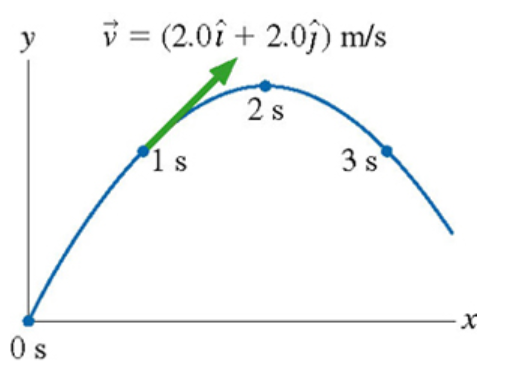# Problem: A physics student on Planet Exidor throws a ball, and it follows the parabolic trajectory shown in the figure. The ball's position is shown at 1s intervals until t=3s. At t=0s , the ball's velocity is v = (2.0 i + 4.0 j) m/s.What was the ball's launch angle?

###### FREE Expert Solution

Two-dimensional vector direction:

$\overline{){\mathbf{tan}}{\mathbit{\theta }}{\mathbf{=}}\frac{{\mathbit{A}}_{\mathbit{y}}}{{\mathbit{A}}_{\mathbit{x}}}}$

81% (396 ratings)###### Problem Details

A physics student on Planet Exidor throws a ball, and it follows the parabolic trajectory shown in the figure. The ball's position is shown at 1s intervals until t=3s. At t=0s , the ball's velocity is v = (2.0 i + 4.0 j) m/s.

What was the ball's launch angle?